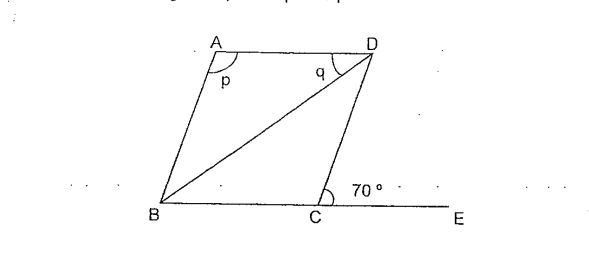# Question 1 of 26

In the figure below, not drawn to scale, ABCD is a rhombus. Given that BCE is a straight line, Find $\angle$p + $\angle$q.A
135$^\circ$
B
140$^\circ$
C
145$^\circ$
D
150$^\circ$
E
None of the above